# [JAVA] I tried to make Venn diagram an easy-to-understand GIF animation

## AND circuit

The AND circuit is as follows. There are inputs A and B, and the overlapping red C is the output.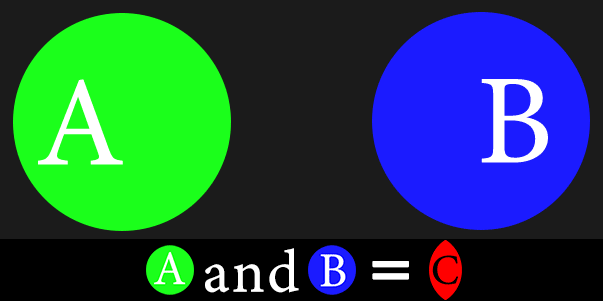## OR circuit

The OR circuit is as follows. There are inputs A and B, and the range C of A and B is the output.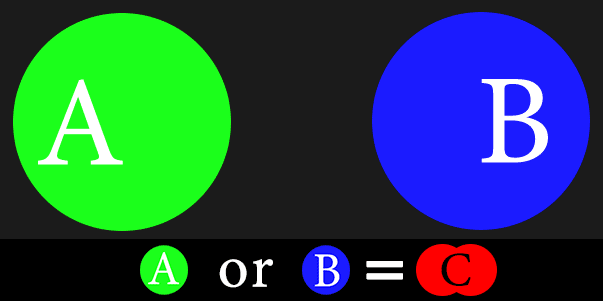## NAND circuit

The NAND circuit looks like this: It is the denial of AND that came out at the beginning. (NOT AND) You can see that the output of red C is the inversion of AND. This circuit outputs FALSE when both A and B are TRUE.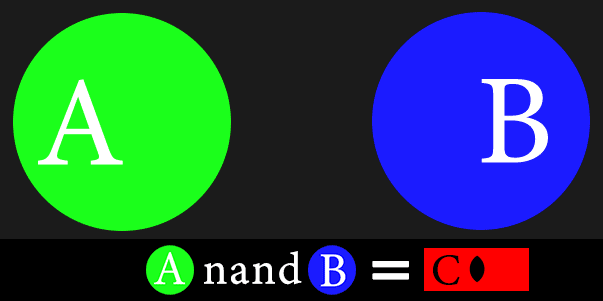## NOR circuit

The NOR circuit is as follows. It is a denial of OR. (NOT OR)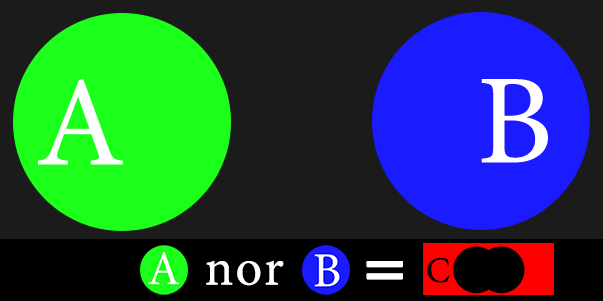## XOR circuit

The XOR circuit looks like this: Outputs FALSE when both A and B are TRUE or both are FALSE.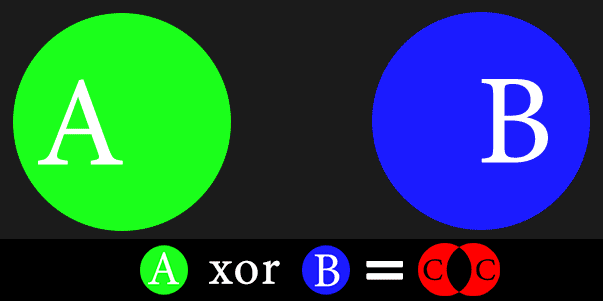## Disassembly of XOR circuit

To find the XOR circuit using NAND, OR, AND, it is as follows. When the yellow NAND and purple OR are overlapped, the overlapping range (AND) is the XOR. (The range where yellow and purple are overlapped and become whitish)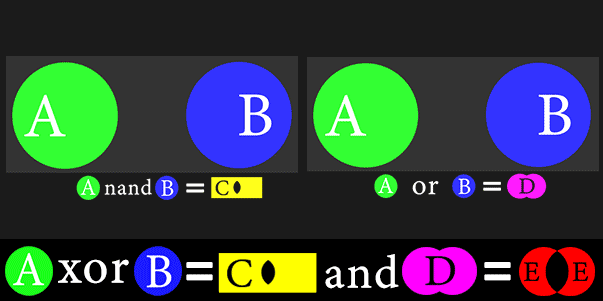## Java implementation of XOR circuit

Let's implement the figure shown in the decomposition of the XOR circuit above in Java.

#### `Main.java`

``````

public class Main {

public static void main(String[] args) {
//XOR circuit output
final boolean[][] AB = {
{ true, true },
{ true, false },
{ false, true },
{ false, false }
};

System.out.printf("XOR circuit output\n");
for (int i = 0; i < AB.length; i++) {
boolean b = xor(AB[i], AB[i]);
System.out.println("(A :" + AB[i] + ") + (B :" + AB[i] + ") = " + b);
}
}
public static boolean and(boolean a, boolean b) {
if (a && b) {
return true;
}
return false;
}

public static boolean or(boolean a, boolean b) {
if (a || b) {
return true;
}
return false;
}
public static boolean nand(boolean a, boolean b) {
return !and(a, b);
}
public static boolean xor(boolean a, boolean b) {
if (and(nand(a, b), or(a,b))) {
return true;
}
return false;
}
}

//XOR circuit output
//(A :true) + (B :true) = false
//(A :true) + (B :false) = true
//(A :false) + (B :true) = true
//(A :false) + (B :false) = false
``````

## Java implementation of XOR circuit Part 2

You can also create an XOR circuit by changing the contents of the xor method used in the above Java code to the following. Below is an output like a crescent moon with the right side of a taking the and of a and nand (a, b) missing, and a crescent moon with the left side of b taking the and of b and nand (a, b) missing. Output so that the output is combined with or.

#### `Main.java`

``````

public static boolean xor(boolean a, boolean b) {
if (or(and(a, nand(a, b)), and(b, nand(a, b)))) {
return true;
}
return false;
}
``````